Select Page

# CBSE Maths 12 Science MCQ Application of Derivatives Answers in English

CBSE Maths 12 Science MCQ Application of Derivatives Answers in English to enable students to get Answers in a narrative video format for the specific question.

Expert Teacher provides CBSE Maths 12 Science MCQ Application of Derivatives Answers through Video Answers in English language. This video solution will be useful for students to understand how to write an answer in exam in order to score more marks. This teacher uses a narrative style for a question from Application of Derivatives not only to explain the proper method of answering question, but deriving right answer too.

Please find the question below and view the Answer in a narrative video format.

Question:

Answer Video in English:

You can select video Answers from other languages also. Please check Answers in ( Hindi )

## Similar Questions from CBSE, 12th Science, Maths, Application of Derivatives

Question 1 : The slope of the normal to the curve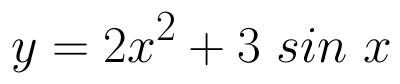at x = 0 is: (View Answer Video)

Question 2 : What is the maximum value of the function sin x+ cos x? (View Answer Video)

Question 3 : Find two positive numbers x  and y such that x+y=60 and is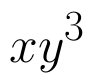maximum. (View Answer Video)

Question 4 : The line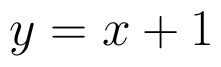is a tangent to the curve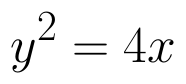at the point. (View Answer Video)

Question 5 : A balloon, which always remains spherical on inflation, is being inflated by pumping in 900 cubic centimetres of gas per second. Find the rate at which the radius of the balloon increases when the radius is 15 cm. (View Answer Video)

### Application of Integrals

Question 1 : Find the area of the circle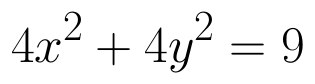which is interior to the parabola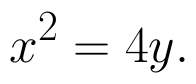(View Answer Video)

Question 2 :

Find the area of the given curves and given lines: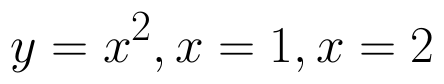and x-axis

Question 3 : Find the area of smaller region bounded by the ellipse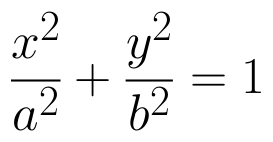and the line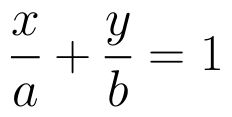. (View Answer Video)

Question 4 : Using integration, find the area bounded by the tangent to the curve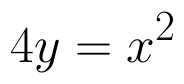at the point (2, 1) and the lines whose equations are x = 2y and x = 3y - 3. (View Answer Video)

Question 5 : Given that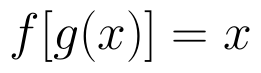, for x = 0 to x = 20. Find f(x) and g(x) such that the area between f(x) and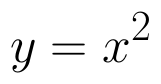from x = 0 to x = 5 be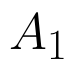and area between g(x) and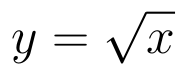from y = 0 to y = 5 be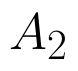. Is? Like functions f and g which work is better, team work or individual work? (View Answer Video)

### Linear Programming

Question 1 : The objective function is maximum or minimum, which lies on the boundary of the feasible region. (View Answer Video)

### Integrals

Question 1 : Find: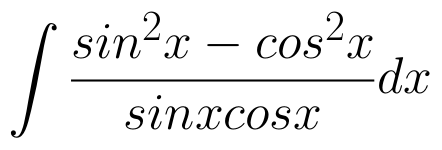Question 2 : Evaluate :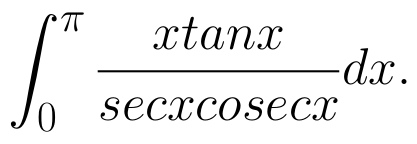(View Answer Video)
Question 3 : Given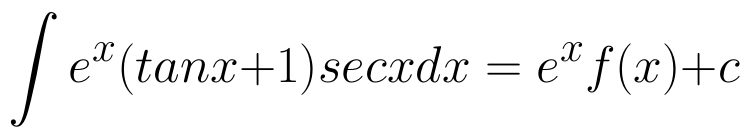. Write f(x) satisfying the above.  (View Answer Video)
Question 4 : Evaluate :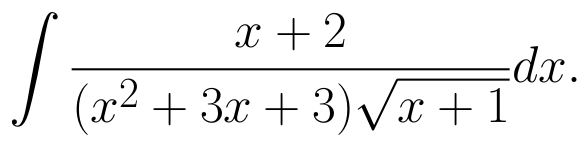(View Answer Video)
Question 5 :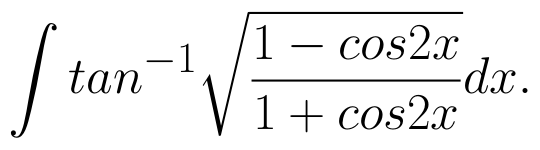(View Answer Video)